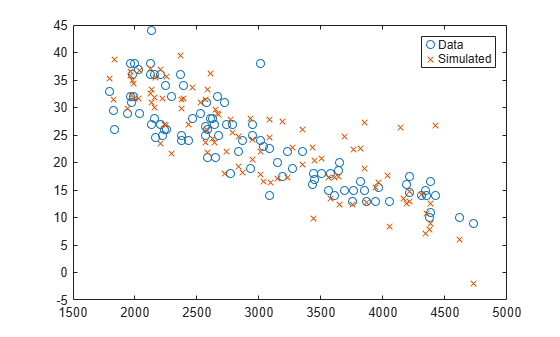random

Class: NonLinearModel

Simulate responses for nonlinear regression model

Syntax

ysim = random(mdl)
ysim = random(mdl,Xnew)
ysim = random(mdl,Xnew,'Weights',W)

Description

ysim = random(mdl) simulates responses from the fitted nonlinear model mdl at the original design points.

ysim = random(mdl,Xnew) simulates responses from the fitted nonlinear model mdl to the data in Xnew, adding random noise.

ysim = random(mdl,Xnew,'Weights',W) simulates responses using the observation weights, W.

Input Arguments

 mdl Nonlinear regression model, constructed by fitnlm. Xnew Points at which mdl predicts responses. If Xnew is a table or dataset array, it must contain the predictor names in mdl.If Xnew is a numeric matrix, it must have the same number of variables (columns) as was used to create mdl. Furthermore, all variables used in creating mdl must be numeric. W Vector of real, positive value weights or a function handle. If you specify a vector, then it must have the same number of elements as the number of observations (or rows) in Xnew.If you specify a function handle, the function must accept a vector of predicted response values as input, and returns a vector of real positive weights as output. Given weights, W, random estimates the error variance at observation i by MSE*(1/W(i)), where MSE is the mean squared error. Default: No weights

Output Arguments

 ysim Vector of predicted mean values at Xnew, perturbed by random noise. The noise is independent, normally distributed, with mean zero, and variance equal to the estimated error variance of the model.

Examples

expand all

Create a nonlinear model of car mileage as a function of weight, and simulate the response.

Create an exponential model of car mileage as a function of weight from the carsmall data. Scale the weight by a factor of 1000 so all the variables are roughly equal in size.

X = Weight;
y = MPG;
modelfun = 'y ~ b1 + b2*exp(-b3*x/1000)';
beta0 = [1 1 1];
mdl = fitnlm(X,y,modelfun,beta0);

Create simulated responses to the data.

Xnew = X;
ysim = random(mdl,Xnew);

Plot the original responses and the simulated responses to see how they differ.

plot(X,y,'o',X,ysim,'x')
legend('Data','Simulated')Alternatives

For predictions without added noise, use predict.